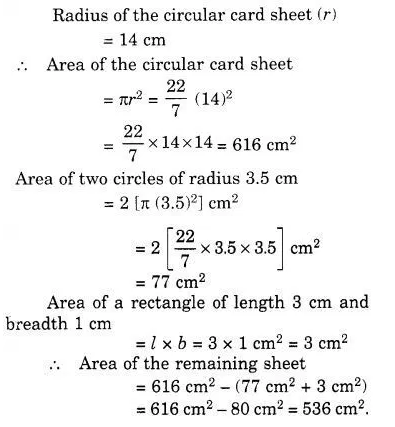# From a circular card sheet of radius 14 cm, two circles of radius 3.5 cm

From a circular card sheet of radius 14 cm, two circles of radius 3.5 cm and a rectangle of length 3 cm and breadth 1 cm are removed (as shown in following figure). Find the area of the remaining sheet. ( Take π =$\frac { 22 }{ 7 }$)